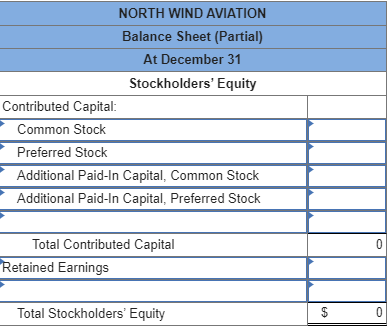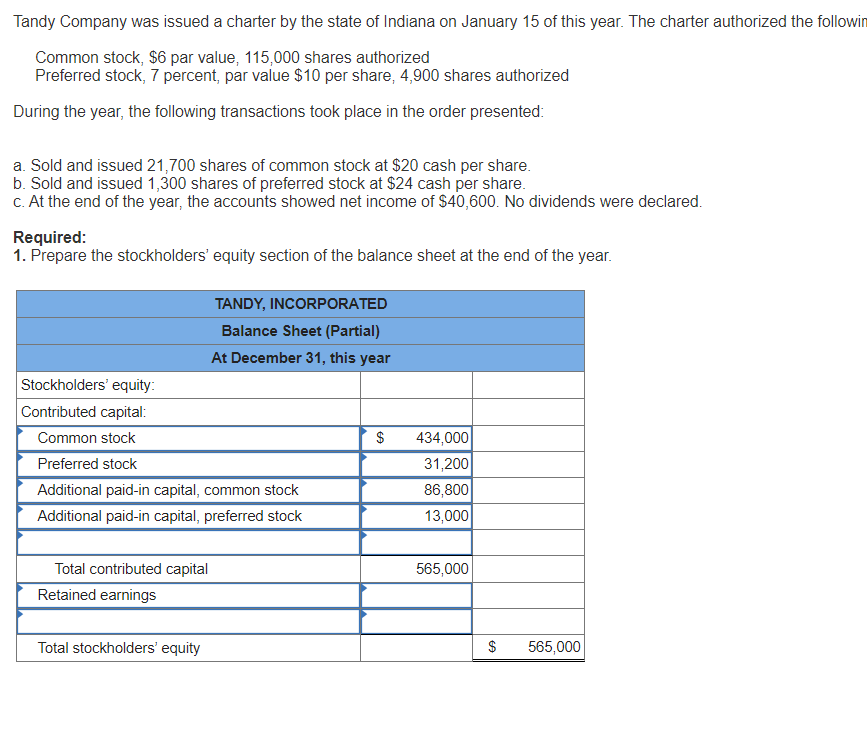Question

# North Wind Aviation received its charter during January authorizing the following capital stock: Preferred stock: 8...

North Wind Aviation received its charter during January authorizing the following capital stock:

Preferred stock: 8 percent, par $10, authorized 20,000 shares. Common stock: par$1, authorized 50,000 shares.

The following transactions occurred during the first year of operations in the order given:

a. Issued a total of 40,000 shares of the common stock for $15 per share b. Issued 10,000 shares of the preferred stock at$16 per share.

c. Issued 3,000 shares of the common stock at $20 per share and 1,000 shares of the preferred stock at$16.

d. Net income for the first year was $48,000, but no dividends were declared. Required: Prepare the stockholders' equity section of the balance sheet at December 31.#### Homework Answers Answer #1 Stockholder’s Equity Stockholder equity refers to the portion of the balance sheet which involves the capital ascertained from the public by issuing them stocks. It also depicts the equity stake on the books currently held by the equity investor of the firm. Thus, the value of the Stockholder’s equity is ascertained by subtracting total liabilities from the total assets. Therefore, computation of the Stockholder’s equity section of the balance sheet for the year-end December 31 is shown below:  Stockholders’ Equity—December 31 Contributed Capital Amount Preferred Stock$110,000 Add: Preferred shares, Additional Capital $66,000 Common Stock$43,000 Add: Common shares, Additional Capital $617,000 Total capital contributed$836,000 Add: Retained Earnings $48,000 Total Stockholder equity$884,000

Hence, it is ascertained that the total stockholder equity accounts for $$\ 884,000$$

Preferred stock

Issued preferred stocks are 11,000 at $$\ 16$$ per share. The par value of the share is $$\ 10$$. The value of outstanding shares is $$\ 110,000(11,000 \times \ 10)$$. The Additional paid in capital of preferred stock are $$\ 66,000$$ is as follows:

\begin{aligned} \text { Additional paid in capital } &=[(\ 16-\ 10 \text { par }) \times 10,000]+[(\ 16-\ 10 \text { par }) \times 1,000] \\ &=(\ 6 \times 10,000)+(\ 6 \times 1,000) \\ &=\ 66,000 \end{aligned}

Common stock

Issued Common stocks are 43,000 at $$\ 15$$ and $$\ 20$$ per share. The par value of the share is $$\ 1$$.

The value of outstanding shares accounts for $$\ 43,000(43,000 \times \ 1)$$. Therefore the computation of the additional paid in capital of common stock are $$\ 617,000$$ is shown below:

\begin{aligned} \text { Additional paid in capital } &=[(\ 15-\ 1 \text { par }) \times 40,000]+[(\ 20-\ 1 \text { par }) \times 3,000] \\ &=(\ 14 \times 40,000)+(\ 19 \times 3,000) \\ &=\ 617,000 \end{aligned}

#### Earn Coins

Coins can be redeemed for fabulous gifts.

Similar Homework Help Questions

• ### Whyville Corporation obtained its charter from the state in January that authorized 500,000 shares of common...

Whyville Corporation obtained its charter from the state in January that authorized 500,000 shares of common stock, $1 par value. During the first year, the company earned$61,000, declared no dividends, and the following selected transactions occurred in the order given: Issued 230,000 shares of the common stock at $26 cash per share. Reacquired 5,300 shares at$27 cash per share to use as stock incentives for senior management. Required information [The following information applies to the questions displayed below.]...

• ### Tandy Company was issued a charter by the state of Indiana on January 15 of this...Tandy Company was issued a charter by the state of Indiana on January 15 of this year. The charter authorized the followin Common stock, $6 par value, 115,000 shares authorized Preferred stock, 7 percent, par value$10 per share, 4,900 shares authorized During the year, the following transactions took place in the order presented: a. Sold and issued 21,700 shares of common stock at $20 cash per share. b. Sold and issued 1,300 shares of preferred stock at$24 cash...